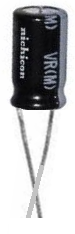﻿ How to Discharge a Capacitor

# How to Discharge a Capacitor

In this article, we will go over the ways in which a capacitor can be discharged.

To discharge a capacitor, the two leads of the capacitor must be connected together so that there is a path for the current to drain through so the capacitor becomes depleted of its power.

The quickest way to discharge a capacitor is to touch the two terminals of the capacitor together, as is shown below.Again, this is the quickest way to discharge a capacitor. However, it's only advised that you do this for capacitors storing a very low voltage. Shorting the terminals of high-voltage capacitors would be very dangerous, because the current created would be very large, and the person would risk the chance of being burnt or electrocuted, which can cause injury or death.

So the other way to discharge a capacitor is to do so through a load, usually a resistor, as such:The value of the resistor depends on the size of the voltage the capacitor is storing. The larger the voltage that it stores, the greater the value of a resistor you should use. If you are working with a very large voltage, you definitely want to use a resistor of several megaohms. This is because if the resistance of the circuit is small and the voltage is great, a large current will be generated, which can be very dangerous. The larger the resistance used, the smaller the current will be, as current is inversely proportional to resistance by Ohm's Law.

Although using a resistor and a large one especially increases the time it takes for a capacitor to discharge, it is a crucial safety measure to make sure that no dangeorous situation occurs.

These are the ways of discharging a capacitor.

Related Resources